Courses

# Differential Equations - 19

## 20 Questions MCQ Test Topic-wise Tests & Solved Examples for IIT JAM Mathematics | Differential Equations - 19

Description
This mock test of Differential Equations - 19 for Mathematics helps you for every Mathematics entrance exam. This contains 20 Multiple Choice Questions for Mathematics Differential Equations - 19 (mcq) to study with solutions a complete question bank. The solved questions answers in this Differential Equations - 19 quiz give you a good mix of easy questions and tough questions. Mathematics students definitely take this Differential Equations - 19 exercise for a better result in the exam. You can find other Differential Equations - 19 extra questions, long questions & short questions for Mathematics on EduRev as well by searching above.
QUESTION: 1

Solution:
QUESTION: 2

### The general solution of the differential equation (y - px) (p  - 1) = p is given by

Solution:

Proof: The given differential equation is
(y - px) (p - i) = p ...... (i)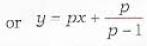......(ii)
Differentiating (ii) w.r. t. x, we get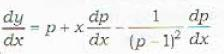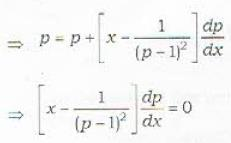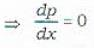⇒ p = c     .......(iii)
Eliminating p from equations (ii) and (iii),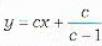QUESTION: 3

### The general solution of i he differential equation sin(y - px) = p is given by

Solution:
QUESTION: 4

The differential equation e3x(p - 1) + p3 e2y = 0 can be reduced to Clairaut’s form by means of the substitution

Solution:

Proof : The given differential equation is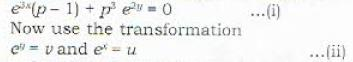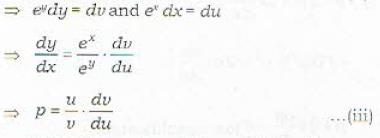Substituting from (ii) and (iii) in (i), we get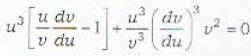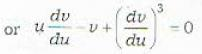or v = up + p2  ......(iv)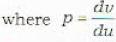Thus equation (iv) is in Ciairaut's form.

QUESTION: 5

Which of the following differential equations cannot be transformed to the Clairaut’s form by means of the substitution x2 = u and y2 = v ?

Solution:

The differential equation in statement (d) can not be reduced to Ciairaut’s form by the transformation
x2 = u and y= v    ....(i)
Proof : The transform ation (i) yields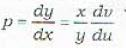.....(ii)
Substitute in the given differential equation from (i) and (ii) and find that the transformed equation is not in Ciairaut’s form.

QUESTION: 6

The differential equation x2p2 + yp(2x + y) + y2 = 0 can be reduced to the Clairaut’s form by means of which of the following substitutions?

Solution:

Hint : The transform aticn xy = v and y = u
implies that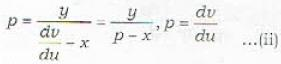The given equation will be reduced to
v = up + p2
which is in the Clairaut's form.

QUESTION: 7

Which of the following statements is incorrect regarding the singular solutions of the differential equations?

Solution:
QUESTION: 8

To obtain the singular solution of the differential equation in Clairaut’s form it is necessary to calculate

Solution:

If the given differential equation is in Ciairaut's form, then any one of the p and c discriminants can be used for finding the singular solution of the differential equation.
Remark : The equation in Clairaut’s form is
y = xp +f(p)    ...(i)
Its general solution is given by
y = cx + f(d)    ...(ii)
Differentiating (ii) partially w.r. t. c,
0 = x + f'(c)       ...(iii)
The singular solution which is the envelope of (ii) can now be obtained by eliminating c from (ii) and (iii).
Alternately : Differentiate (i) partially w.r. t. p,
0 = x + f'(P)    ...(iv)
The singular solution can now be obtained by eliminating p from (i) and (iv).

QUESTION: 9

The singular solution (s) of the differential equation 4xp2 = (3x - a)2 is/arc given by

Solution:
QUESTION: 10

The differential equation p2 + y2 = 1 has how many singular solutions?

Solution:

Proof : The given differential equation is P + = 1 ...(i)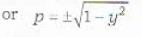...(iii)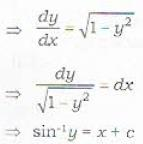⇒ y = sin (x + c) ...(iii)
Differentiating (iii) w.r.t. c, we get 0 = cos (x + c)
⇒ x + c - π/2 ...(iv)
Substituting for x + c from (iv) in (iii), one singular solution is given by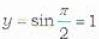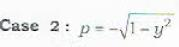This gives another singular solution as y = -1.
∴ There are 2 singular solutions
Note : complete case - 2.

QUESTION: 11

The differential equation p2(1 - x2) = 1 - y2 has how many singular solutions?

Solution:

Obtain all the four singular solutions.

QUESTION: 12

The singular solution(s) of the differential equation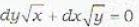is/are given by

Solution:

Proof : The given differential equation is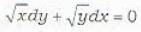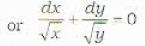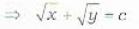......(i)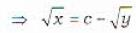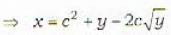...(ii)
Differentiating (ii)partially with respect to c, we get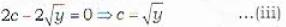Substituting for c from (iii) in (i), one singular solution is given by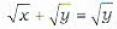or x = 0
Similarly, if we rewrite (i) as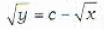then another singular solution will be y = 0
∴ x = 0 arid y = 0
are both singular solutions.

QUESTION: 13

The singular solution(s) of the differential equation 8ap3 = 27y is/are given by

Solution:
QUESTION: 14

The general solution of the differential equation (a2 - x2)p2 + 2xyp + (b2 - y2) = 0 represents a family of straight lines whose envelope is the

Solution:
QUESTION: 15

The Euler’s method provides the solution of which of the following firsi order differential equations

Solution:

Euler's method provided the solution of all I he first order differential equations of the form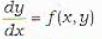QUESTION: 16

The Euler’s method for solving a differential equation of the type dy/dx = f(x, y) provides

Solution:

Euler’s method.
This method provides the solution of the differential equations of the form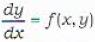in the form of a set of tabulated values. This method is very slow and to obtain reasonable accuracy with Euler's method, h should be taken very small. This method provid es an approximate solution of equation (i) in general.

QUESTION: 17

The solution of the differential equation dy/dx = f(x, y) through a given point (x0, y0) can be written as y = F(x). The Euler's method determines discrete points on the solution curve y = F(x). Let (x1 , y1,) be first point calculated and let (y')0 denote the value of dy/dx at (x0, y0) then

Solution:

Proof : Integrating the differential equation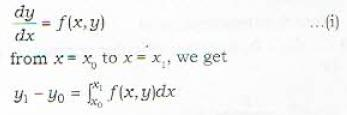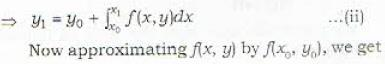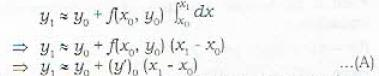QUESTION: 18

Let (x2, y2) be the second point calculated by the Euler’s method on the solution curve y = F(x). Then

Solution:
QUESTION: 19

Let the solution of the differential equation dy/dx = x + y pass through (0, 1) and let (0.05, y1) be the first point calculated on the solution curve by the Euler’s method. Then y1 is equal to

Solution:
QUESTION: 20

Consider the differential equation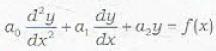This will be differential equation with constant coefficients if

Solution:

If the coefficients a0, a1 and a2 are constants in the differential eauation.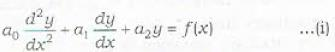then it will be adifferential equation with constant coefficients.
Remark : There is no conditon on f(x) and it may be a constant (zero or non-zero) or a function of x.